# Extended interpolation process

(diff) ← Older revision | Latest revision (diff) | Newer revision → (diff)

An interpolation process constructed from a given interpolation process by imposing additional interpolation conditions.

## The method of additional nodes.

The method of additional nodes was introduced by J. Szabados in [a4] to approximate the derivatives of a function by means of the derivative of the Lagrange interpolating polynomial (cf. also Lagrange interpolation formula). For given interpolation nodes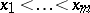, the method consists in considering the interpolation process with respect to the nodeswhere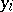,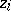are equidistant nodes betweenand, respectively between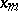and.

For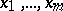being the zeros of the Jacobi polynomial (cf. Jacobi polynomials)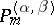,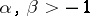, the Lebesgue constants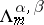of the Lagrange interpolating polynomialshave the behaviour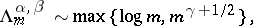where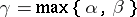. Therefore, only if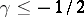willhave the optimal behaviour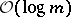. Denoting by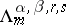the Lebesgue constant of the extended interpolation process, one has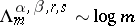if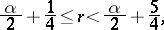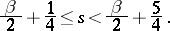This technique has been extended to more general contexts and has led to the construction of many classes of optimal interpolation processes (see [a1], [a3] and the literature cited therein). These results have given important contributions to numerical quadrature and to collocation methods in the numerical solution of functional equations.

## Error estimation.

An efficient method for the practical estimation of the error of an interpolation process with respect to given nodesconsists in imposing interpolation conditions at suitable additional nodes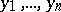. In particular, a natural choice areand nodes which interlace,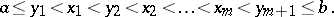Letbe the zeros of the orthogonal polynomials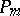with respect to some weight function. The zeros of the orthogonal polynomials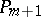with respect to the same weight functionhave the interlacing property; orthogonal polynomials with respect to other weight functions have also been considered. For necessary and sufficient conditions for the convergence of the extended interpolation process, cf. [a2] and the literature cited therein. The zeros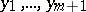of the associated Stieltjes polynomials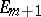lead to the Lagrange–Kronrod formulas, and they maximize the algebraic degree of the corresponding interpolatory quadrature formulas; the latter are the Gauss–Kronrod quadrature formula. For the zeros of the Stieltjes polynomials, the interlacing property does not hold for general, but it is known for several important weight functions including the Legendre weight (see Stieltjes polynomials). Error estimation by extended interpolation is an important tool for the numerical approximation of linear functionals.

How to Cite This Entry:
Extended interpolation process. Encyclopedia of Mathematics. URL: http://encyclopediaofmath.org/index.php?title=Extended_interpolation_process&oldid=13703
This article was adapted from an original article by Sven EhrichG. Mastroianni (originator), which appeared in Encyclopedia of Mathematics - ISBN 1402006098. See original article